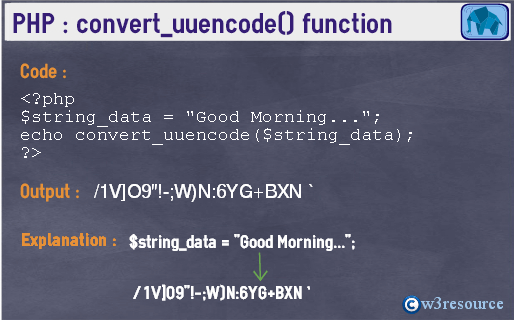# PHP : convert_uuencode() function

## Description

The convert_uuencode() function is used to encode a uuencoded string.

Version

(PHP 5)

Syntax

`convert_uuencode(string_data)`

Parameter

Name Description Required /
Optional
Type
string_data The uuencoded data. Required String

Return values

Returns the uuencoded data

Value Type: String.

Pictorial PresentationExample:

``````<?php
\$string_data = "Good Morning...";
echo convert_uuencode(\$string_data);
?>``````

Output:

`/1V]O9"!-;W)N:6YG+BXN`

View the example in the browser

See also

PHP Function Reference

Previous: convert_uudecode
Next: count_chars

﻿

## PHP: Tips of the Day

Float

Example:

```\$float = 0.123;
```

For historical reasons "double" is returned by gettype() in case of a float, and not simply "float"

Floats are floating point numbers, which allow more output precision than plain integers.

Floats and integers can be used together due to PHP's loose casting of variable types:

```\$sum = 3 + 0.14;
echo \$sum;
```

Output:

```3.14
```

php does not show float as float number like other languages, for example:

```\$var = 1;
echo ((float) \$var);
```

Output:

```1
```